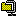사이트 사이의 인증 공유

2008.01.01 13:26
이 문서는 여러 사이트에서 인증 정보를 간단하게 공유하는 방법을 설명하고 있습니다. 만약 협력 관계에 있는 다른 사이트와 로그인 정보 등을 간단하고 안전하게 공유해야 한다면, 이 문서의 내용이 조금은 도움이 될 거 같습니다.
예제는 ASP, ASP.NET 1.1, ASP.NET 2.0, JSP, PHP 5 용으로 총 5개가 작성되었으며, 문서는 워드 2003으로 작성되었습니다.

참고로 암호화를 할 때 임의 문자열(salt)과 클라이언트 IP를 덧붙이고, 복호화 후 클라이언트 IP 검사를 추가하면 보안성을 더 높일 수 있습니다.

여러 웹 프로그래밍 언어에서 서로 호환되는 문자열 암호화 클래스

2007.12.19 17:31StringEncrypter.zip

소스 관리의 편의 문제로 현재 주석은 영어로 작성되어 있습니다.
(ASP 와 ASP.NET 용 새 버전이 있습니다.)

ASP, ASP.NET 1.1/2.0, JSP, PHP 5 에서 사용할 수 있는 문자열 암호화 클래스를 만들어 봤습니다. 이 클래스들은 서로 호환되기 때문에 ASP에서 암호화한 문자열을 ASP.NET 에서 복호화하는 식으로 사용이 가능합니다. 이 클래스는 초기화 벡터는 문자열로 입력받습니다. 내부적으로 키와 초기화 벡터는 UTF-8으로 변환된 후, MD5로 해시됩니다.문자열 암호화는 Encrypt 메서드에서 수행합니다. 이 메서드는 먼저 입력 문자열을 UTF-8으로 변환한 후, PKCS7 패딩을 덧붙입니다. (JSP 버전에서는 PKCS5를 사용하지만, 블록 크기가 128비트일 경우에는 서로 호환됩니다.) 그 다음에 128-bit AES 알고리즘을 사용하여 CBC 모드로 암호화합니다. 암호화된 이진 데이터는 베이스64로 인코딩됩니다.
문자열 복호화에는 Decrypt 메서드를 사용합니다.다음 코드는 ASP.NET 1.1/2.0(C#)에서 문자열 암호화 클래스를 사용하는 예제입니다. StringEncrypter 클래스는 StringEncrypter.cs 파일에 구현되어 있습니다.
```/*
*  ASP.NET 1.1/2.0 (C#)
*/
using Hyeongryeol.Security.Cryptography ;
...
const string key = "암호화 비밀키입니다." ;
const string iv = "초기화 벡터입니다." ;

// 인스턴스 만들기.
StringEncrypter encrypter = new StringEncrypter (key, iv) ;

// 문자열 암호화.
string encrypted = encrypter.Encrypt ("테스트 문자열") ;

// 문자열 복호화.
string decrypted = encrypter.Decrypt (encrypted) ;
```
다음 코드는 ASP(VBScript)에서 문자열 암호화 클래스를 사용하는 예제입니다. ASP는 자체적으로 AES 알고리즘을 지원하지 않기 때문에, COM 컴포넌트인 HyeongryeolStringEncrypter.dll 파일을 regsvr32.exe 프로그램으로 먼저 설치해야 합니다. AES 알고리즘은 XySSL 0.8을 기반으로 합니다.
(설치는 %WINDOWS%System32 폴더에 할 것을 권장합니다.)
```'
'  ASP (VBScript)
'
Const conKey = "암호화 비밀키입니다."
Const conIV = "초기화 벡터입니다."

' 인스턴스 만들기.
Set objEncrypter = Server.CreateObject ("Hyeongryeol.StringEncrypter")

objEncrypter.Key = conKey
objEncrypter.InitialVector = conIV

' 문자열 암호화.
strEncrypted = objEncrypter.Encrypt ("테스트 문자열")

' 문자열 복호화.
strDecrypted = objEncrypter.Decrypt (strEncrypted)
```
다음 코드는 JSP (자바)에서의 예제입니다. StringEncrypter 클래스는 StringEncrypter.java 파일에 구현되어 있습니다. 이 클래스를 사용하려면 Base64Encoder.java 파일도 있어야 합니다.
```/*
*  JSP (Java)
*/
import com.hyeongryeol.security.cryptography.*;
...
String key = "암호화 비밀키입니다.";
String iv = "초기화 벡터입니다.";

// 인스턴스 만들기.
StringEncrypter encrypter = new StringEncrypter(key, iv);

// 문자열 암호화.
String encrypted = encrypter.encrypt("테스트 문자열");

// 문자열 복호화.
String decrypted = encrypter.decrypt(encrypted);
```
다음 코드는 PHP 5에서의 예제입니다. StringEncrypter 클래스는 StringEncrypter.php 파일에 구현되어 있습니다. 이 클래스는 UTF-8 형식으로 저장된 파일에서만 사용할 수 있습니다. (클래스에서는 UTF-8 인코딩과 디코딩을 하지 않습니다.)
```/*
*  PHP 5 (UTF-8)
*/
require ("StringEncrypter.php") ;

define ("KEY", "암호화 비밀키입니다.") ;
define ("IV", "초기화 벡터입니다.") ;

// 인스턴스 만들기.
\$encrypter = new StringEncrypter (KEY, IV) ;

// 문자열 암호화.
\$encrypted = \$encrypter->encrypt ("테스트 문자열") ;

// 문자열 복호화.
\$decrypted = \$encrypter->decrypt (\$encrypted) ;
```

참고자료

Interoperable string encrypters for the web.

2007.12.19 16:01StringEncrypter.zip

A new version is available for ASP and ASP.NET.

I've written string encrypters for ASP, ASP.NET 1.1/2.0, JSP and PHP 5 respectivly, which are interoperable with each other.
The encrypters take two strings, a key and an initial vector. The key and the initial vector are internally converted into UTF-8 and hashed by MD5.String encryption is carried out by using Encrypt method, which first converts the input string into UTF-8 and encodes it according to the PKCS7 padding algorithm (except JSP that uses PKCS5, but it is compatible if the block size is 128bit.) and encrypts it with 128-bit AES algorithm in Cipher Block Chaining(CBC). The encrypted binary is encoded by Base64.
Decrypt method decrypts the encrypted string.The following code is an example of using the string encrypter in ASP.NET 1.1/2.0(C#). The StringEncrypter class is implemented in StringEncrypter.cs.
```/*
*  ASP.NET 1.1/2.0 (C#)
*/
using Hyeongryeol.Security.Cryptography ;
...
const string key = "This is a secret key." ;
const string iv = "This is an initial vector." ;

// Create an instance.
StringEncrypter encrypter = new StringEncrypter (key, iv) ;

// Encrypt a string.
string encrypted = encrypter.Encrypt ("Test sample") ;

// Decrypt a string.
string decrypted = encrypter.Decrypt (encrypted) ;
```
The following code shows how to use the string encrypter in ASP(VBScript). Since ASP doesn't support built-in AES algorithm, you need to install HyeongryeolStringEncrypter.dll which is a COM component based on XySSL 0.8. You have to register it by using the regsvr32.exe program.
(I recommend you to install it in the %WINDOWS%System32 folder.)
```'
'  ASP (VBScript)
'
Const conKey = "This is a secret key."
Const conIV = "This is an initial vector."

' Create an instance.
Set objEncrypter = Server.CreateObject ("Hyeongryeol.StringEncrypter")

objEncrypter.Key = conKey
objEncrypter.InitialVector = conIV

' Encrypt a string.
strEncrypted = objEncrypter.Encrypt ("Test sample")

' Decrypt a string.
strDecrypted = objEncrypter.Decrypt (strEncrypted)
```
The following code is an example in JSP (Java). The StringEncrypter class is implemented in StringEncrypter.java which also requires Base64Encoder.java.
```/*
*  JSP (Java)
*/
import com.hyeongryeol.security.cryptography.*;
...
String key = "This is a secret key.";
String iv = "This is an initial vector.";

// Create an instance.
StringEncrypter encrypter = new StringEncrypter(key, iv);

// Encrypt a string.
String encrypted = encrypter.encrypt("Test sample");

// Decrypt a string.
String decrypted = encrypter.decrypt(encrypted);
```
The following code is an example in PHP 5. The StringEncrypter class is implemented in StringEncrypter.php. It can only be used in a UTF-8 encoded file because UTF-8 encoding and decoding are omitted.
```/*
*  PHP 5 (UTF-8)
*/
require ("StringEncrypter.php") ;

define ("KEY", "This is a secret key.") ;
define ("IV", "This is an initial vector.") ;

// Create an instance.
\$encrypter = new StringEncrypter (KEY, IV) ;

// Encrypt a string.
\$encrypted = \$encrypter->encrypt ("Test sample") ;

// Decrypt a string.
\$decrypted = \$encrypter->decrypt (\$encrypted) ;
```

References